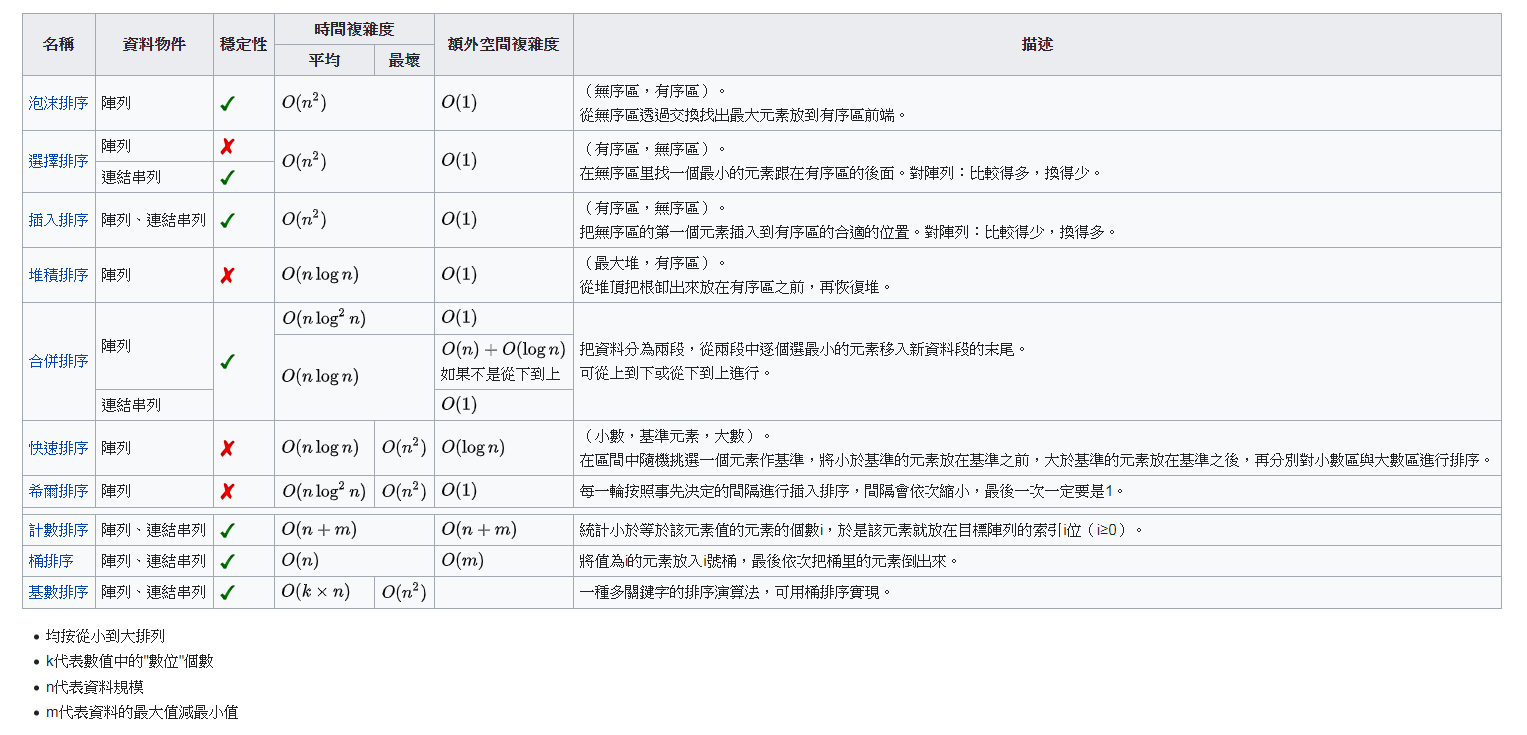## J.J.'s Blogs

Like Share Discussion Bookmark Smile

# 排序演算法 | 簡介

• 輸出結果為遞增序列（遞增是針對所需的排序順序而言）
• 輸出結果是原輸入的一種排列、或是重組

# 分類

• 計算的時間複雜度（最差、平均、和最好表現），依據串列（list）的大小(n)。一般而言，好的表現是O(n log n)(大O符號)，壞的表現是O(n²)。對於一個排序理想的表現是O(n)，但平均而言不可能達到。基於比較的排序演算法對大多數輸入而言至少需要O(n log n)。
• 記憶體使用量（以及其他電腦資源的使用）
• 穩定性：穩定排序演算法會讓原本有相等鍵值的紀錄維持相對次序。也就是如果一個排序演算法是穩定的，當有兩個相等鍵值的紀錄R和S，且在原本的串列中R出現在S之前，在排序過的串列中R也將會是在S之前。
• 依據排序的方法：插入、交換、選擇、合併等等。

# 排序演算法列表

• 穩定的排序

• 泡沫排序（bubble sort）— O(n²)
• 插入排序（insertion sort）—O(n²)
• 雞尾酒排序（cocktail sort）—O(n²)
• 桶排序（bucket sort）—O(n)；需要O(k)額外空間
• 計數排序（counting sort）—O(n+k)；需要O(n+k)額外空間
• 合併排序（merge sort）—O(n log n)；需要O(n)額外空間
• 原地合併排序— O(n log ² n)如果使用最佳的現在版本
• 二元排序樹排序（binary tree sort）— O(n log n)期望時間；O(n²)最壞時間；需要O(n)額外空間
• 鴿巢排序（pigeonhole sort）—O(n+k)；需要O(k)額外空間
• 侏儒排序（gnome sort）— O(n²)
• 圖書館排序（library sort）— O(n log n)期望時間；O(n²)最壞時間；需要(1+𝓔)n額外空間
• 塊排序（block sort）— O(n log n)
• 不穩定的排序

• 選擇排序（selection sort）—O(n²)
• 希爾排序（shell sort）—O(n log² n)如果使用最佳的現在版本
• 克洛弗排序（Clover sort）—O(n)期望時間，O(n²)最壞情況[來源請求]
• 梳排序— O(n log n)
• 堆積排序（heap sort）—O(n log n)
• 平滑排序（smooth sort）— O(n log n)
• 快速排序（quick sort）—O(n log n)期望時間，O(n²)最壞情況；對於大的、亂數串列一般相信是最快的已知排序
• 內省排序（introsort）—O(n log n)
• 耐心排序（patience sort）—O(n log n+k)最壞情況時間，需要額外的O(n+k)空間，也需要找 - 到最長的遞增子序列（longest increasing subsequence）
• 不實用的排序

• Bogo排序— O(n × n!)}O(n × n!)}，最壞的情況下期望時間為無窮。
• Stupid排序—O(n³);遞迴版本需要O(n²)額外記憶體
• 珠排序（bead sort）— O(n) 或 O(∫n),但需要特別的硬體
• 煎餅排序—O(n),但需要特別的硬體
• 臭皮匠排序（stooge sort）演算法簡單，但需要約n².⁷的時間

# 簡要比較